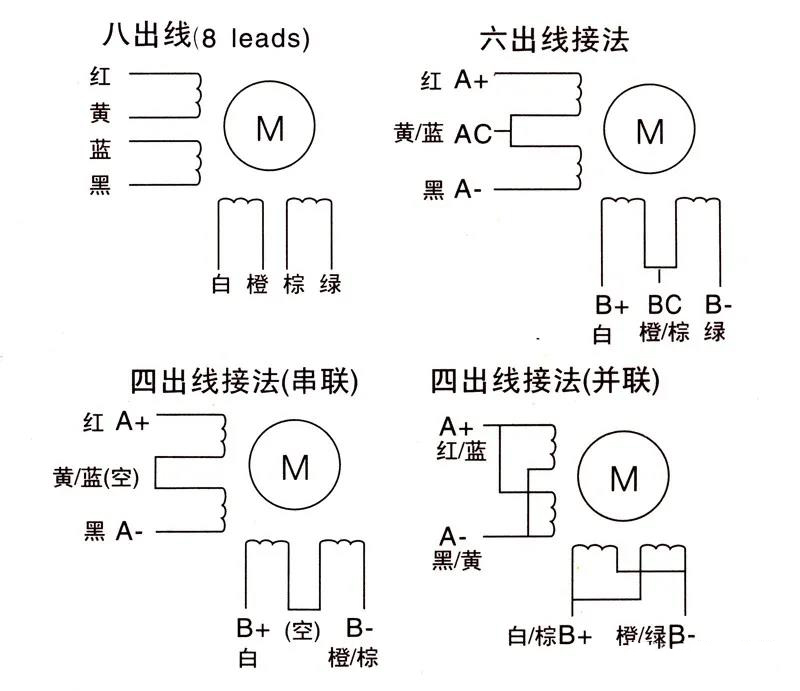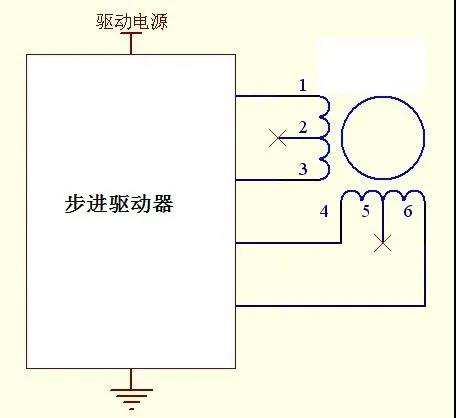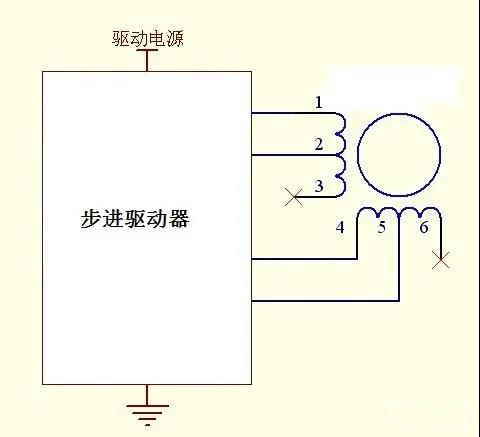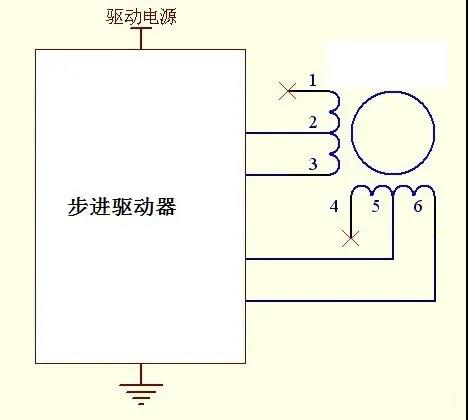You are here: / / How the two phase stepper motor connects wire?

# How the two phase stepper motor connects wire?

Views:2     Author:Site Editor     Publish Time: 2020-11-11      Origin:Site

The two-phase stepper motor usually has three types: four, six, and eight wire. The schematic diagram is as follows:In reality, the two-phase stepper motor comes from four phases. The four-wire type is drawn in series or parallel and then draws four wires. In terms of six wire types, one is drawn from the middle of the A-phase and B phase to form six wires. There is no internal connection in eight wire, which is directly drawn from eight wire of four phases. When in use, you can connect according to your needs.

Four-wire connection: the simplest connection is four-wire type, as long as you can determine which two are A phase and which two are B phase. There is no need to determine the negative or positive. By using a buzzer multimeter to test any two wires, the two conduction are in a group. The short joint rotation method can also be sued, if the motor has resistance, then the two short ends are in a group. You can determine any one group is A phase, others are B phase. If the direction of the motor is opposite, then switch the two groups.Six-wire connection: using a multimeter to determine which three are a group, according to a formula that the resistance between the middle and any two is half of the resistance between the other two. The most used type is:Eight-wire connection: this type should be combined into four types and then connected to the driver. When combined, it can be in series and parallel. The combination method can refer to a four-wire connection diagram. We can use the same method in four-wire type to judge which four types are, however, it is hard to determine which two groups can be combined into one group. We can use arbitrary two groups to respectively connect the A+/A and B+/B-. sending the pulse to the driver, if the motor can rotate, then the two chosen groups are not in the same phase. If the motor can not rotate, the two chosen groups are in the same phase. Keeping A+/A- remain and then removing B+/B- to switch another group, until finding a group that can rotate. Recording the connection color of B+/B- and the rotation direction of the motor. If the rotation direction two times is the same, then B+ polarity is the same, the B- polarity is the same. If the direction is opposite, the B+ in the first time and B- in the second time is the same polarity, the B- in the first time and B+ in the second time are the same polarity.Low speed, high torque in series. High speed, low torque in parallel:

Parallel connection: The resistance and inductance of the winding are reduced by multiples. The acceleration performance of the motor is good. However, the required current is twice the rated current, the heating is relatively high, which has a higher requirement on the output capacity of the driver.

Series connection: the resistance and inductance of windings are increased by multiples. The motor runs stably at low speed, the noise, and the heat is low, which does not have a higher requirement on the driver. But at high speed, the loss of torque is large.

Conclusion:

The four-wire connection is simple, but flexibility is poor. Whether choosing parallel or series connections, it should base on the application. Six-wire and eight-wire can switch according to their needs, which is very flexible.